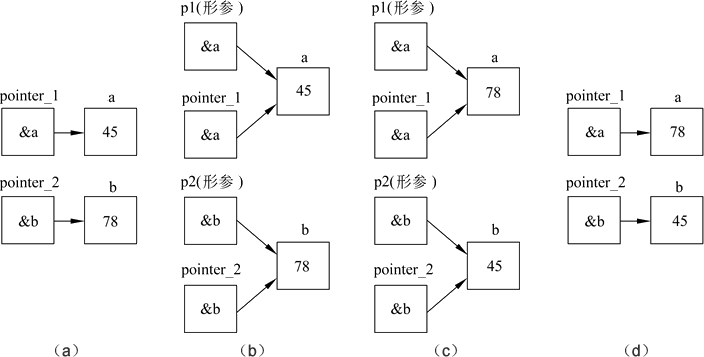# C++指针作为函数参数

【例6.3】题目同例6.2，即对输入的两个整数按大小顺序输出。这里用函数处理，而且用指针类型的数据作函数参数。程序如下：

1. #include <iostream>
2. using namespace std;
3. int main( )
4. {
5. void swap(int *p1,int *p2); //函数声明
6. int *pointer_1,*pointer_2,a,b; //定义指针变量pointer_1,pointer_2,整型变量a,b
7. cin>>a>>b;
8. pointer_1=&a; //使pointer_1指向a
9. pointer_2=&b; //使pointer_2指向b
10. if(a<b) swap(pointer_1,pointer_2); //如果a<b,使*pointer_1和*pointer_2互换
11. cout<<"max="<<a<<" min="<<b<<endl; //a已是大数,b是小数
12. return 0;
13. }
14.
15. void swap(int *p1,int *p2) //函数的作用是将*p1的值与*p2的值交换
16. {
17. int temp;
18. temp=*p1;
19. *p1=*p2;
20. *p2=temp;
21. }

45 78↙
max=78 min=45

if(a<b) swap(*pointer_1, *pointer_2);1. void swap(int *p1, int *p2)
2. {
3. int *temp;
4. *temp=*p1; //此语句有问题
5. *p1=*p2;
6. *p2=*temp;
7. }

1. void swap(int x, int y)
2. {
3. int temp;
4. temp=x;
5. x=y;
6. y=temp;
7. }1. 在主调函数中设n个变量，用n个指针变量指向它们；
2. 编写被调用函数，其形参为n个指针变量，这些形参指针变量应当与主调函数中的n个指针变量具有相同的基类型；
3. 在主调函数中将n个指针变量作实参，将它们的值(是地址值)传给所调用函数的n个形参指针变量，这样，形参指针变量也指向这n个变量；
4. 通过形参指针变量的指向，改变该n个变量的值;
5. 在主调函数中就可以使用这些改变了值的变量。

1. #include <iostream>
2. using namespace std;
3. int main( )
4. {
5. void swap(int *p1,int *p2);
6. int *pointer_1,*pointer_2,a,b;
7. cin>>a>>b;
8. pointer_1=&a;
9. pointer_2=&b;
10. if(a<b) swap(pointer_1,pointer_2);
11. cout<<"max="<<a<<" min="<<b<<endl;
12. return 0;
13. }
14.
15. void swap(int *p1,int *p2)
16. {
17. int *temp;
18. temp=p1;
19. p1=p2;
20. p2=temp;
21. }【例6.4】输入a，b，c 3个整数，按由大到小的顺序输出。

1. #include <iostream>
2. using namespace std;
3. int main( )
4. {
5. void exchange(int *,int *,int *); //对exchange函数的声明
6. int a,b,c,*p1,*p2,*p3;
7. cin>>a>>b>>c; //输入3个整数
8. p1=&a;p2=&b;p3=&c; //指向3个整型变量
9. exchange(p1,p2,p3); //交换p1,p2,p3指向的3个整型变量的值
10. cout<<a<<" "<<b<<" "<<c<<endl; //按由大到小的顺序输出3个整数
11. }
12.
13. void exchange(int *q1,int *q2,int *q3)
14. {
15. void swap(int *,int *); //对swap函数的声明
16. if(*q1<*q2) swap(q1,q2); //调用swap,将q1与q2所指向的变量的值互换
17. if(*q1<*q3) swap(q1,q3); //调用swap,将q1与q3所指向的变量的值互换
18. if(*q2<*q3) swap(q2,q3); //调用swap,将q2与q3所指向的变量的值互换
19. }
20.
21. void swap(int *pt1,int *pt2) //将pt1与pt2所指向的变量的值互换
22. {
23. int temp;
24. temp=*pt1;
25. *pt1=*pt2;
26. *pt2=temp;
27. }

12 -56 87↙
87 12 -56

(2)
100%

(0)
0%